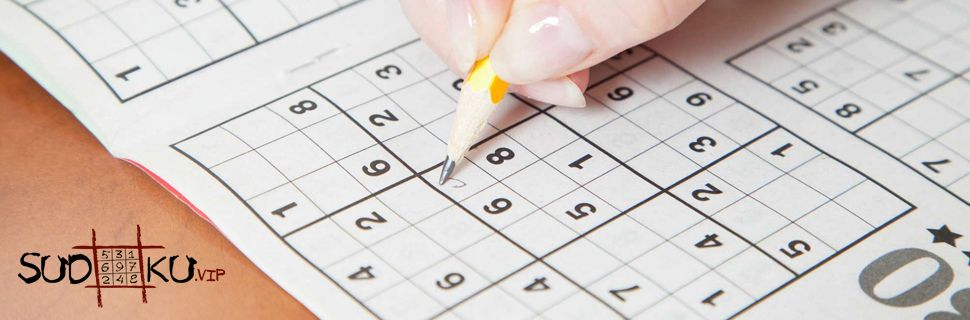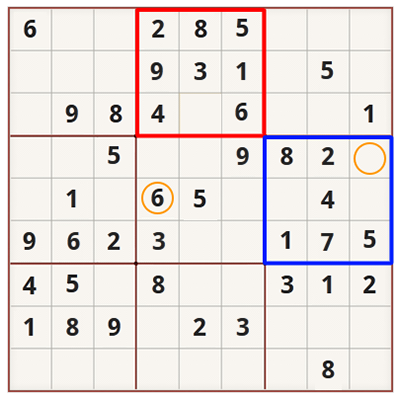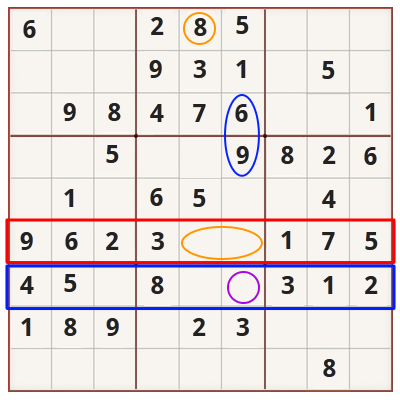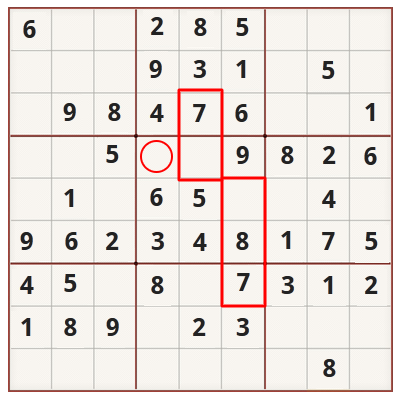# Sudoku strategy for beginnersFortunately, Sudoku is not that difficult of a game as it seems at first sight. If you know how to approach the Sudoku grid and find few shortcuts, soon you’ll learn to recognize the patterns in the game and you’ll find out the possibilities of placing the numbers in the right place.

Here are some advices for beginners:

First look for easy options

Every Sudoku grid, when starting the game, comes with few numbers placed on their right place. This can give you a good start to complete the puzzle. When starting a new game, watch carefully where most numbers are placed in a row, column or square. Sometimes the grid will do half of the work itself!For an example, in this puzzle, the square marked in red already has 8 out of 9 numbers. That makes it easier in placing the missing 9th number by checking which number is missing. In this case the missing number is 7. Now we know which number to place without doing anything else.

In the meantime, when we take a look at the right square marked in blue, we see that are missing 3 numbers- 3,6 and 9.

When we look at the square left of it, we notice the number 6 (circled in orange) in the central row - which means that the two empty spaces in the central row on the blue square can’t contain the number 6, so there is only one cell remaining where we can place it. The upper right cell circled in orange is the place where we should place number 6.

Observe the nearby rows

Sudoku is a game of model recognition and opportunity finding. Overtime you’ll get better and faster in finding and eliminating certain numbers, but for those Sudoku beginners, the best strategy is to observe the nearby rows and columns.

For an example, in the grid below, the red row has 2 empty spaces (enclosed in orange). After checking the numbers in the red row, we know that are missing the numbers 8 and 4. If we look to the top we’ll see where the numbers should be, there is 8 in the middle column (enclosed in orange).Now we already know that the left empty space can’t contain the number 8 – so we have to place 4, which lead us to place 8 on the right empty space.

Also, if we look at the blue row we see that 6 of 9 spaces are filled. After checking which numbers we have, we can see that are missing 6, 9 and 7. If we look above, we’ll spot that 6 and 9 are engaged in the column where is the right empty space from the row (circled in purple). By eliminating the possible choices, we know that we can’t place 6 or 9, so there should be the number 7.

Trace the moment

Sudoku is a momentum game – every time you place a new number, try to trace what had changed and what other numbers you can fill. With each added number the grid changes, opening up new opportunities.

For example, in this grid, the central square has only 3 empty spaces, which remain unfilled and we know that we can place numbers 1,2 and 7.As we described in the tips above, we already had placed 7 in the square below the central one, at the upper right corner and in the top row of the central square (enclosed in red).

That means we can eliminate the number 7 from the empty spaces, finding out that we have to place 7 in the top left corner of the central square (enclosed in red circle).

After we place 7, we can eliminate the numbers 1 and 2 – and if we look at the right column, we’ll see that we already have 1, which means that the right empty space can’t contain the number 1. The simple process of elimination will allow us to finish the central square - and every new number we place in this square influences its accompanying squares, rows and columns. Each number placed on its rightful place brings us closer to successfully solving the puzzle.